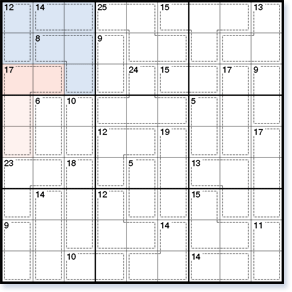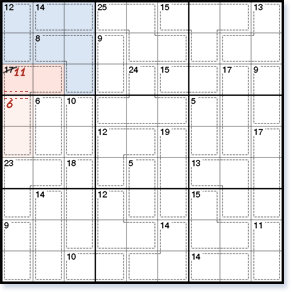# Killer Sudoku solving techniques

Killer Sudoku adds a new dimension to standard Sudoku, requiring arithmetic to solve. You will need specialised Killer Sudoku solving techniques to progress in these puzzles besides the standard Sudoku techniques.

## Multiple Square 45 Rule

The multiple square 45 rule is an extension of the 45 rule. It applies the 45 rule when there is more than one inside or outside square.Figure 1

In the top left block (highlighted in Fig. 1), all cages lie completely inside with the exception of the cage with total 17 in the first and second columns. This 17 cage has two squares inside the block, and two squares outside. You can therefore use the multiple square 45 rule to find the total of the two inside squares and of the two outside squares. Unlike the standard 45 rule, you will end up with the total of two squares, instead of the solution for one square.

The inside total for the top left block is 12 + 14 + 8 = 34. So the total of the two inside squares is 45 – 34 = 11, and the total of the two outside squares is 17 – 11 = 6.

Using this information, you can now split the 17 cage up into two smaller cages, each with its own total, as shown in Fig. 2.Figure 2

Although this method has not immediately solved any squares, it has given you more information about the squares in the 17 cage which will be useful later on in the solving process. Each smaller split cage will have fewer possible combinations than the larger 17 cage. The split cage with total 11 can contain the numbers {29}, {38}, {47} or {56} and the split cage with total 6 can be either {15} or {24}.

In general, look for a row, columns or block where all cages except one lie completely inside, and this cage has multiple squares both inside and outside.